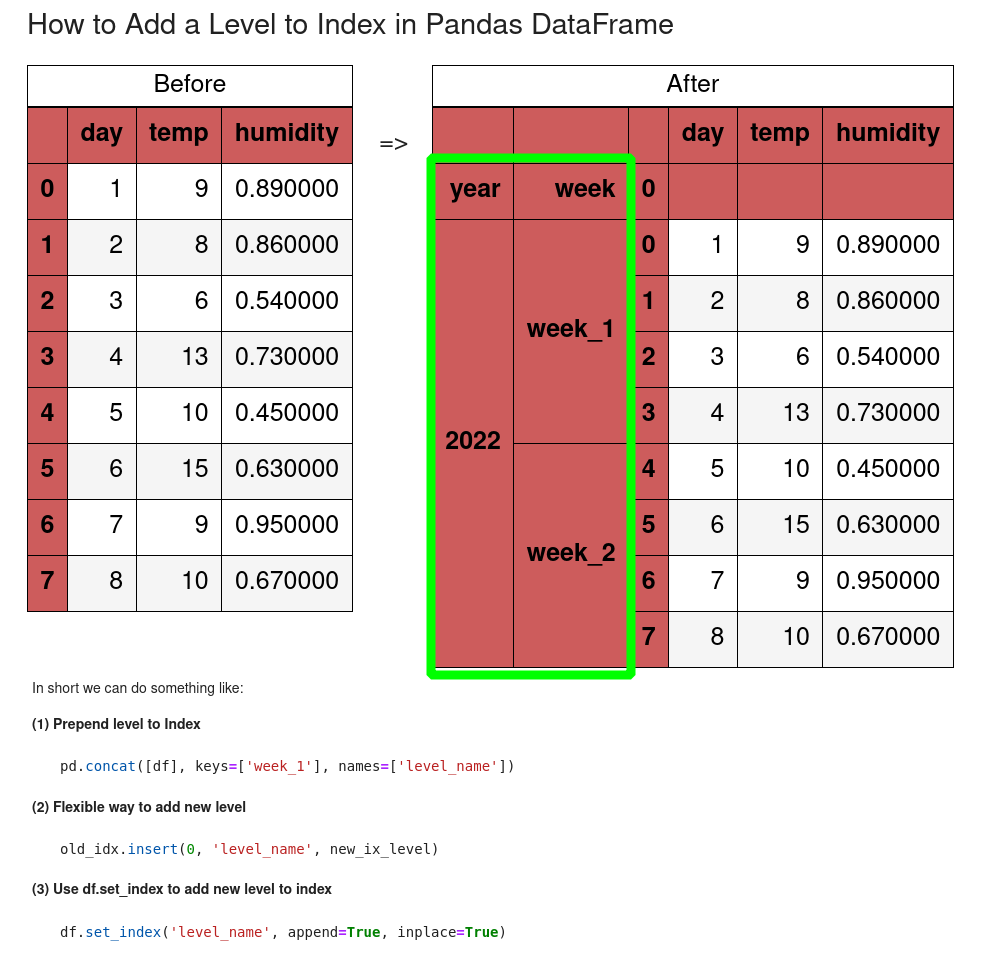In this tutorial, we'll explore how to add level to MultiIndex in Pandas DataFrame.

We will learn how to add levels to rows or columns.

In short we can do something like:

(1) Prepend level to Index

``````pd.concat([df], keys=['week_1'], names=['level_name'])
``````

(2) Flexible way to add new level

``````old_idx.insert(0, 'level_name', new_ix_level)
``````

(3) Use df.set_index to add new level to index

``````df.set_index('level_name', append=True, inplace=True)
``````

## Setup

Let's work with the following DataFrame:

``````import pandas as pd

data = {'day': [1, 2, 3, 4, 5, 6, 7, 8],
'temp': [9, 8, 6, 13, 10, 15, 9, 10],
'humidity': [0.89, 0.86, 0.54, 0.73, 0.45, 0.63, 0.95, 0.67]}

df = pd.DataFrame(data=data)
``````

data looks like:

day temp humidity
0 1 9 0.89
1 2 8 0.86
2 3 6 0.54
3 4 13 0.73
4 5 10 0.45

## 1: Add first level to Index/MultiIndex (rows)

Let's start by adding new level to index - so we will create MultiIndex from index in Pandas DataFrame:

``````pd.concat([df], keys=['week_1'], names=['level_name'])
``````

Before this operation we have - `df.index`:

``````RangeIndex(start=0, stop=8, step=1)
``````

After this operation we get:

``````MultiIndex([('week_1', 0),
('week_1', 1),
('week_1', 2),
('week_1', 3)...],
names=['level_name', None])
``````

The new DataFrame with the new MultiIndex look like:

day temp humidity
level_name
week_1 0 1 9 0.89
1 2 8 0.86
2 3 6 0.54
3 4 13 0.73
4 5 10 0.45

### Access level of MultiIndex

Pro Tip 1
To access the data from the multiIndex we can do:
`df.loc[('week_1', 0)]`

This will result into:

``````day         1.00
temp        9.00
humidity    0.89
Name: (week_1, 0), dtype: float64
``````

## 2: Add second level to Index/MultiIndex (rows)

Alternatively we can add second(inner) level to create MultiIndex by:

• creating new column with values
• set the new column as index
``````df['level_name'] = 'week_1'
df.set_index('level_name', append=True, inplace=True)
``````

The result is:

day temp humidity
level_name
0 week_1 1 9 0.89
1 week_1 2 8 0.86
2 week_1 3 6 0.54
3 week_1 4 13 0.73
4 week_1 5 10 0.45

This time the DataFrame MultiIndex is:

``````MultiIndex([(0, 'week_1'),
(1, 'week_1'),
(2, 'week_1'),
(3, 'week_1')..],
names=[None, 'level_name'])
``````

## 3: Add first level to columns(index)

To add new level to the columns and create a MultiIndex we can use the following code:

``````df = pd.concat([df], keys=['week_1'], names=['level_name'], axis=1)
``````

This will change our DataFrame to:

level_name meteo
day temp humidity
0 1 9 0.89
1 2 8 0.86
2 3 6 0.54
3 4 13 0.73
4 5 10 0.45

Checking the column index - `df.columns`:

``````MultiIndex([('meteo',      'day'),
('meteo',     'temp'),
('meteo', 'humidity')],
names=['level_name', None])
``````## 4: Flexible way to add new level of Index (rows/columns)

A generic solution to add new levels of Index or MultiIndex in Pandas DataFrame is by:

• converting the index to DataFrame
• update the index
• change back to index/MultiIndex

So the code below shows all the steps:

``````new_ix_level = ['week_1'] * 4 + ['week_2'] * 4 # new values for the level

old_idx = df.index.to_frame() # convert to DataFrame

old_idx.insert(0, 'level_name', new_ix_level) # add new level

df.index = pd.MultiIndex.from_frame(old_idx)
``````

The new level of the MultiIndex has several different values:

``````MultiIndex([('week_1', 0),
('week_1', 1),
('week_1', 2),
('week_1', 3),
('week_2', 4),
('week_2', 5)..],
names=['level_name', 0])
``````

and the DataFrame is:

day temp humidity
0 1 9 0.89
1 2 8 0.86
2 3 6 0.54
3 4 13 0.73
4 5 10 0.45

## 5: Add multiple levels to index

Finally let's check how we can add multiple levels to index in Pandas DataFrame.

We will use the last generic solution in order to add two levels to the index - this will create MultiIndex with 3 levels:

``````new_ix_level = ['week_1'] * 4 + ['week_2'] * 4
new_ix_level_1 = ['2022'] * 8

old_idx = df.index.to_frame()

old_idx.insert(0, 'week', new_ix_level)
old_idx.insert(0, 'year', new_ix_level_1)

df.index = pd.MultiIndex.from_frame(old_idx)
``````

result:

day temp humidity
year week 0
2022 week_1 0 1 9 0.89
1 2 8 0.86
2 3 6 0.54
3 4 13 0.73
week_2 4 5 10 0.45

## Conclusion

In this post, we covered multiple ways to add levels to Index or MultiIndex in Pandas DataFrame.

We saw how to add the first or last level and a generic solution for adding different values - in the new level. Finally we also saw how to add multiple levels at once.